CAT  >  Test: Quadratic Equations- 5

Test Description

## 15 Questions MCQ Test Quantitative Aptitude (Quant) | Test: Quadratic Equations- 5

Test: Quadratic Equations- 5 for CAT 2023 is part of Quantitative Aptitude (Quant) preparation. The Test: Quadratic Equations- 5 questions and answers have been prepared according to the CAT exam syllabus.The Test: Quadratic Equations- 5 MCQs are made for CAT 2023 Exam. Find important definitions, questions, notes, meanings, examples, exercises, MCQs and online tests for Test: Quadratic Equations- 5 below.
Solutions of Test: Quadratic Equations- 5 questions in English are available as part of our Quantitative Aptitude (Quant) for CAT & Test: Quadratic Equations- 5 solutions in Hindi for Quantitative Aptitude (Quant) course. Download more important topics, notes, lectures and mock test series for CAT Exam by signing up for free. Attempt Test: Quadratic Equations- 5 | 15 questions in 15 minutes | Mock test for CAT preparation | Free important questions MCQ to study Quantitative Aptitude (Quant) for CAT Exam | Download free PDF with solutions
 1 Crore+ students have signed up on EduRev. Have you?
Test: Quadratic Equations- 5 - Question 1

###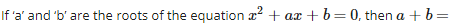Detailed Solution for Test: Quadratic Equations- 5 - Question 1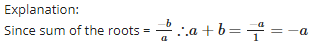Test: Quadratic Equations- 5 - Question 2

### The sum of reciprocals of Sharma’s age 3 years ago and 5 years from now is 1/3, then his present age is

Detailed Solution for Test: Quadratic Equations- 5 - Question 2

Explanation:

Let Sharma’s present age be x years then his age 3 years ago is (x−3) years and 5 years from now is (x+5)years. According to question,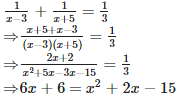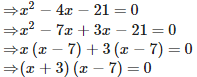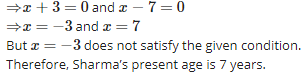Test: Quadratic Equations- 5 - Question 3

### If the quadratic equation kx(x – 2) + 6 = 0 has equal roots, then the value of ‘k’ is

Detailed Solution for Test: Quadratic Equations- 5 - Question 3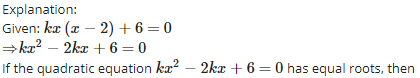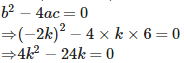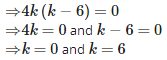Test: Quadratic Equations- 5 - Question 4

If one root of the equation ax2+bx+c=0 is three times the other, then b2:ac=

Detailed Solution for Test: Quadratic Equations- 5 - Question 4

Explanation:

Let one root be αα then other root will be 3α
∴ Sum of the roots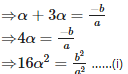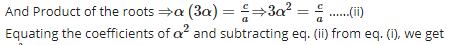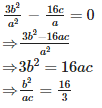Test: Quadratic Equations- 5 - Question 5

A train travels 360km at a uniform speed. If the speed had been 5 km/hr more, it would have taken 1 hour less for the same journey, then the actual speed of the train is

Detailed Solution for Test: Quadratic Equations- 5 - Question 5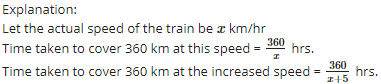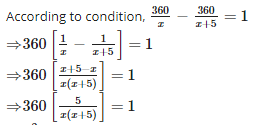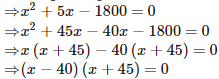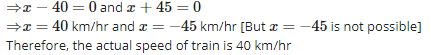Test: Quadratic Equations- 5 - Question 6

Let b = a + c. Then the equation ax2+bx+c=0 has equal roots if

Detailed Solution for Test: Quadratic Equations- 5 - Question 6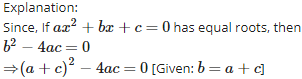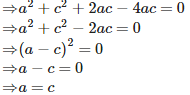Test: Quadratic Equations- 5 - Question 7

The discriminant of the quadratic equation 3√3x2+10x+√3=0

Detailed Solution for Test: Quadratic Equations- 5 - Question 7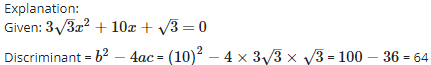Test: Quadratic Equations- 5 - Question 8

If one root of the equation 4x2−2x+(λ−4)=0 be the reciprocal of the other, then the value of λ is

Detailed Solution for Test: Quadratic Equations- 5 - Question 8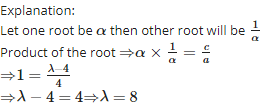Test: Quadratic Equations- 5 - Question 9

Rohan’s mother is 26 years older than him. The product of their ages 3 years from now will be 360, then Rohan’s present age is

Detailed Solution for Test: Quadratic Equations- 5 - Question 9

Explanation:

Let Rohan’s present age be xx years.
Then Rohan’s mother age will be (x+26) years.
And after 3 years their ages will be (x+3) and (x+29) years. According to question,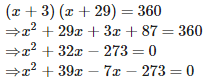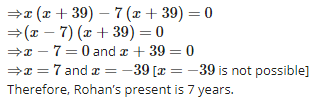Test: Quadratic Equations- 5 - Question 10

The nature of the roots of the equation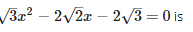Detailed Solution for Test: Quadratic Equations- 5 - Question 10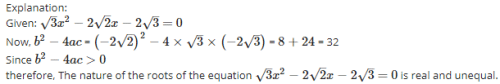Test: Quadratic Equations- 5 - Question 11

By a reduction of Re.1 per kg in the price of sugar, Radha can buy one kg sugar more for Rs.56. The original price of 1 kg of sugar is

Detailed Solution for Test: Quadratic Equations- 5 - Question 11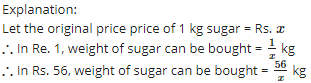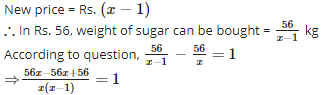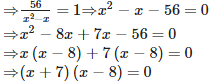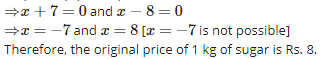Test: Quadratic Equations- 5 - Question 12

If the sum of the roots of x2−(k+6)x+2(2k−1)=0 is equal to half of their product, then k =

Detailed Solution for Test: Quadratic Equations- 5 - Question 12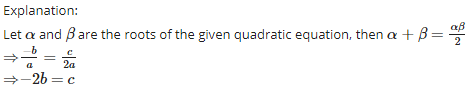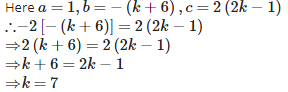Test: Quadratic Equations- 5 - Question 13

500 bananas were divided equally among a certain number of students. If there were 25 more students, each would have received one banana less. Then the number of students is

Detailed Solution for Test: Quadratic Equations- 5 - Question 13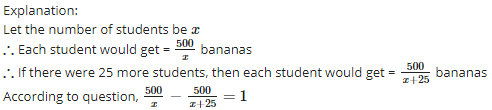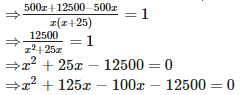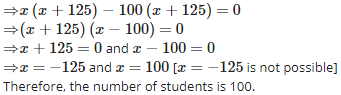Test: Quadratic Equations- 5 - Question 14

A cyclist takes 2 hours less to cover a distance of 200 km, if he increases his speed by 5 km/hr. Then his original speed is

Detailed Solution for Test: Quadratic Equations- 5 - Question 14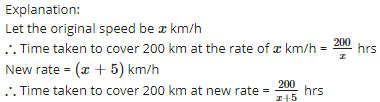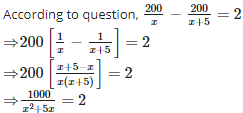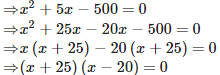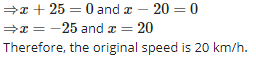Test: Quadratic Equations- 5 - Question 15

The discriminant of 4x2 + 3x – 2 = 0 is

Detailed Solution for Test: Quadratic Equations- 5 - Question 15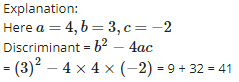## Quantitative Aptitude (Quant)

163 videos|163 docs|131 tests
 Use Code STAYHOME200 and get INR 200 additional OFF Use Coupon Code